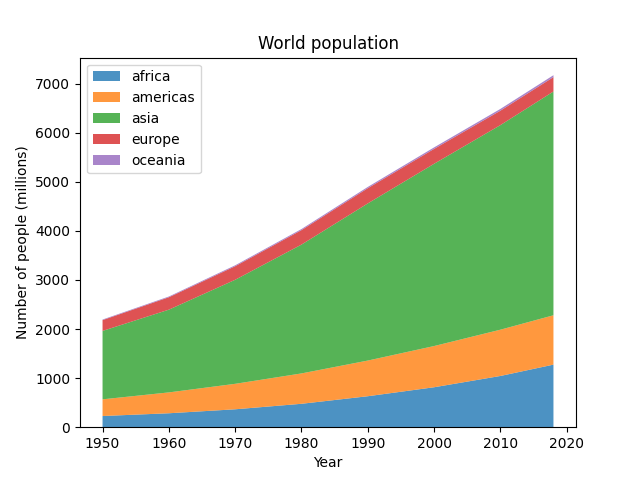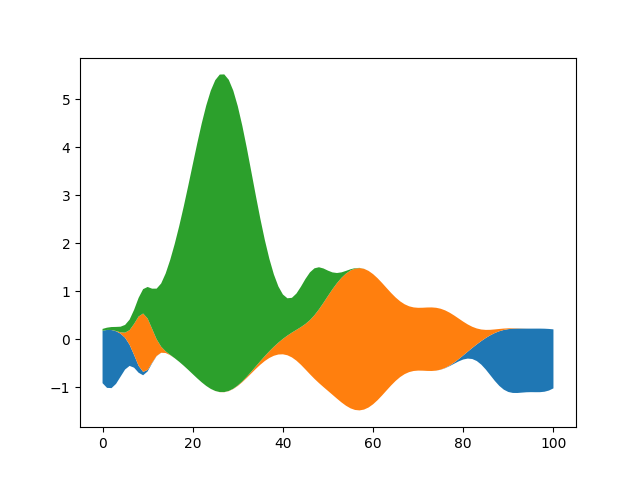# Stackplots and streamgraphs#

## Stackplots#

Stackplots draw multiple datasets as vertically stacked areas. This is useful when the individual data values and additionally their cumulative value are of interest.

import numpy as np
import matplotlib.pyplot as plt

# data from United Nations World Population Prospects (Revision 2019)
# https://population.un.org/wpp/, license: CC BY 3.0 IGO
year = [1950, 1960, 1970, 1980, 1990, 2000, 2010, 2018]
population_by_continent = {
'africa': [228, 284, 365, 477, 631, 814, 1044, 1275],
'americas': [340, 425, 519, 619, 727, 840, 943, 1006],
'asia': [1394, 1686, 2120, 2625, 3202, 3714, 4169, 4560],
'europe': [220, 253, 276, 295, 310, 303, 294, 293],
'oceania': [12, 15, 19, 22, 26, 31, 36, 39],
}

fig, ax = plt.subplots()
ax.stackplot(year, population_by_continent.values(),
labels=population_by_continent.keys(), alpha=0.8)
ax.legend(loc='upper left')
ax.set_title('World population')
ax.set_xlabel('Year')
ax.set_ylabel('Number of people (millions)')

plt.show()## Streamgraphs#

Using the baseline parameter, you can turn an ordinary stacked area plot with baseline 0 into a stream graph.

# Fixing random state for reproducibility
np.random.seed(19680801)

def gaussian_mixture(x, n=5):
"""Return a random mixture of *n* Gaussians, evaluated at positions *x*."""
amplitude = 1 / (.1 + np.random.random())
dx = x[-1] - x
x0 = (2 * np.random.random() - .5) * dx
z = 10 / (.1 + np.random.random()) / dx
a += amplitude * np.exp(-(z * (x - x0))**2)
a = np.zeros_like(x)
for j in range(n):